# Basic Concepts¶

PyTuning is purposefully designed to be as simple as possible, so that non-programmers (musicologists, musicians, etc.) can use it without too much difficulty: the data structures are relatively simple; there are currently no classes defined, instead opting for an imperative/procedural approach.

Regardless of how it is used (interactively or as a development library), the user should have a good understanding of some of the basic, foundational concepts the package uses.

## Scales¶

A scale is, simply, a list of degrees. By convention the list is bookended by the unison and the octave, with each degree given as a frequency ratio relative to the root tone of the scale. The first degree is always, the ratio of the first degree to the first degree. Most commonly the last degree is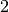, as the octave is usually twice the frequency of the root (although the package has some support for non-standard “octaves”).

As an example, this is the standard 12-tone equal temperament scale (sometimes referred to as 12-TET, or 12-EDO, for Equal Division of the Octave).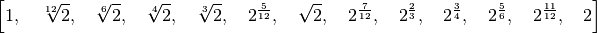A few things to note:

• As mentioned previously, the scale includes the unison and octave.
• Each scale degree is a SymPy number, so it is represented symbolically. Note that algebraic simplifications are performed by default.
• Even though the length of this list of 13, it is considered a 12 note scale, because the unison and the octave is in actuality the same note. Many of the functions in this package ask one to choose the number of notes or degrees to be used in this function. For these you should follow this convention.

(For those who are curious: the generation of scales is documented in Scale Creation, but the above scale was generated with the following code:

from pytuning.scales import create_edo_scale
edo_12_scale = create_edo_scale(12)


Simplified versions of most functions are provided in the interactive environment.)

## Degrees¶

Scale degrees (which are expressed as frequency rations relative to the tonic of the scale) are expressed in SymPy values. In practical terms the Integer and Rational class will be used the most, but SymPy is a full-featured package, and you may benefit from having some familiarity with it.

An example of a few degrees:

import sympy as sp

unison           = sp.Integer(1)                      # Normal unison
octave           = sp.Integer(2)                      # Normal octave
perfect_fifth    = sp.Rational(3,2)                   # As a rational number
minor_second_tet = sp.Integer(2) ** sp.Rational(1,12) # the 12th root of 2
# could also by sp.root(2,12)
lucy_L           = sp.root(2,2*sp.pi)                 # Lucy scale Long step


Will yield the following: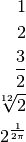SymPy manipulates all values analytically, but sometimes one needs a floating approximation to a degree (for example, tuning a synthesizer usually needs frequencies expressed as floating point numbers). For the evalf() member function can be used:

print(unison.evalf())
1.00000000000000
print(octave.evalf())
2.00000000000000
print(perfect_fifth.evalf())
1.50000000000000
print(minor_second_tet.evalf())
1.05946309435930
print(lucy_L.evalf())
1.11663288009114


## Modes¶

A mode is a selection of notes from a scale, and is itself a list of degrees (and therefore is also a scale). A mode can be produced from a scale by applying a mask to the scale. Again, the functions involved are documented elsewhere, but as example this is how we would produce the standard major scale (which in the context of this package would be referred to as a mode):

major_mask = (0,2,4,5,7,9,11,12)
major_mode = mask_scale(edo_12_scale, major_mask)


which produces the following scale: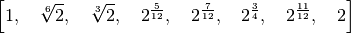## Mode Objects¶

Some functions in this package return a mode object. For example, the find_best_modes() function will take a scale and find a mode (or modes), based upon some consonance metric function. Here is one such object, which is implemented as a Python dict.

{'mask': (0, 2, 3, 5, 7, 9, 10, 12),
'metric_3': 22.1402597402597,
'original_scale': [1,
256/243,
9/8,
32/27,
81/64,
4/3,
1024/729,
3/2,
128/81,
27/16,
16/9,
243/128,
2],
'scale': [1, 9/8, 32/27, 4/3, 3/2, 27/16, 16/9, 2],
'steps': [2, 1, 2, 2, 2, 1, 2],
'sum_distinct_intervals': 12,
'sum_p_q': 161,
'sum_p_q_for_all_intervals': 4374,
'sum_q_for_all_intervals': 1822}


The meaning of these keys:

• original_scale is the original scale which was input into the function. In this example is was a Pythagorean scale.
• scale is the output scale of the function
• mask is the mask of the original scale that produces the output
• steps is similar to mask, but reported in a different format. Each entry in the steps list represents the number of degrees in the original scale between successive degrees in the returned scale. The standard major scale, for example, would be represented by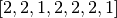.

In this example there are also other keys included. sum_distinct_intervals, sum_p_q, sum_p_q_for_all_intervals, sum_q_for_all_intervals, and metric_3 are the outputs of calculated metric functions. This particular mode, for example, has a rating of 161 by the sum_p_q metric.

Metric functions are describe briefly below, and in more detail in Metric Functions.

## Tuning Tables¶

Tuning Tables are a representation of a scale, usually a string (which can be written to a file), which can be understood by an external software package. As an example, to take a standard Pythagorean scale and produce a representation understood by Scala:

pythag_scale       = create_pythagorean_scale()
scala_tuning_table = create_scala_tuning(pythag_scale, "Pythagorean Scale")


The variable scala_tuning_table now contains the following:

! Scale produced by pytuning. For tuning yoshimi or zynaddsubfx,
! only include the portion below the final '!'
!
Pythagorean Scale
12
!
256/243
9/8
32/27
81/64
4/3
1024/729
3/2
128/81
27/16
16/9
243/128
2/1


For many tuning tables one has to pin the scale to some reference frequency. For this the convention of MIDI note number is employed. For example, in the MIDI standard the note 69 is A 440 Hz, so by specifying a reference of 69, the corresponding entry in the table would be 400 Hz, and this would represent the root or tonic degree of the scale.

Exporting the above scale in a Csound compatible format:

csound_tuning_table = create_csound_tuning(pythag_scale, reference_note=69)


yields the following:

f1 0 256 -2     8.14815     8.70117     9.16667     9.65706    10.31250    10.86420    11.60156    12.22222 \
13.05176    13.75000    14.48560    15.46875    16.29630    17.40234    18.33333    19.31413 \
20.62500    21.72840    23.20313    24.44444    26.10352    27.50000    28.97119    30.93750 \
32.59259    34.80469    36.66667    38.62826    41.25000    43.45679    46.40625    48.88889 \
52.20703    55.00000    57.94239    61.87500    65.18519    69.60938    73.33333    77.25652 \
82.50000    86.91358    92.81250    97.77778   104.41406   110.00000   115.88477   123.75000 \
130.37037   139.21875   146.66667   154.51303   165.00000   173.82716   185.62500   195.55556 \
208.82813   220.00000   231.76955   247.50000   260.74074   278.43750   293.33333   309.02606 \
330.00000   347.65432   371.25000   391.11111   417.65625   440.00000   463.53909   495.00000 \
521.48148   556.87500   586.66667   618.05213   660.00000   695.30864   742.50000   782.22222 \
835.31250   880.00000   927.07819   990.00000  1042.96296  1113.75000  1173.33333  1236.10425 \
1320.00000  1390.61728  1485.00000  1564.44444  1670.62500  1760.00000  1854.15638  1980.00000 \
2085.92593  2227.50000  2346.66667  2472.20850  2640.00000  2781.23457  2970.00000  3128.88889 \
3341.25000  3520.00000  3708.31276  3960.00000  4171.85185  4455.00000  4693.33333  4944.41701 \
5280.00000  5562.46914  5940.00000  6257.77778  6682.50000  7040.00000  7416.62551  7920.00000 \
8343.70370  8910.00000  9386.66667  9888.83402 10560.00000 11124.93827 11880.00000 12515.55556


This is a 128-entry table, mapping note number to absolute frequency. Csound’s table opcode can be used to index into the table and play the appropriate frequency, using something like the following:

inote     init          p4
iveloc    init          p5
ifreq     table         inote, 1
a1        oscil         iveloc, ifreq, 2
outs          a1, a1


(This assumes that p4 in the orchestra file contains MIDI note numbers, of course. If you use a different convention there are translation opcodes that can be used.)

## Metric Functions¶

Metric Functions are functions that takes a scale as an input and returns a numeric value calculated from that scale. It is used, for example, in find_best_modes() to evaluate the consonance of a scale (find_best_modes() uses a metric to evaluate the consonance of all possible modes of a scale and returns the evaluation of those modes as a mode_object).

The return value of a metric function should be a dict with a unique string identifier as the key and the metric as the value.

As an example, the following is one of the package-defined metrics:

As an example of use, the following:

pythag = create_pythagorean_scale()
metric = sum_p_q_for_all_intervals(pythag)


yields the following:

{'sum_p_q_for_all_intervals': 1092732}